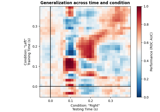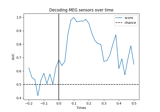# mne.decoding.GeneralizingEstimator#

class mne.decoding.GeneralizingEstimator(base_estimator, scoring=None, n_jobs=None, *, position=0, verbose=None)[source]#

Generalization Light.

Fit a search-light along the last dimension and use them to apply a systematic cross-tasks generalization.

Parameters:
base_estimatorobject

The base estimator to iteratively fit on a subset of the dataset.

scoring

Score function (or loss function) with signature `score_func(y, y_pred, **kwargs)`. Note that the “predict” method is automatically identified if scoring is a string (e.g. `scoring='roc_auc'` calls `predict_proba`), but is not automatically set if `scoring` is a callable (e.g. `scoring=sklearn.metrics.roc_auc_score`).

n_jobs

The number of jobs to run in parallel. If `-1`, it is set to the number of CPU cores. Requires the `joblib` package. `None` (default) is a marker for ‘unset’ that will be interpreted as `n_jobs=1` (sequential execution) unless the call is performed under a `joblib.parallel_backend()` context manager that sets another value for `n_jobs`.

position`int`

The position for the progress bar.

verbose

Control verbosity of the logging output. If `None`, use the default verbosity level. See the logging documentation and `mne.verbose()` for details. Should only be passed as a keyword argument.

Attributes:
classes_

Methods

 Estimate distances of each data slice to all hyperplanes. `fit`(X, y, **fit_params) Fit a series of independent estimators to the dataset. `fit_transform`(X, y, **fit_params) Fit and transform a series of independent estimators to the dataset. `get_params`([deep]) Get parameters for this estimator. Predict each data slice with all possible estimators. Estimate probabilistic estimates of each data slice with all possible estimators. `score`(X, y) Score each of the estimators on the tested dimensions. `set_params`(**params) Set the parameters of this estimator. Transform each data slice with all possible estimators.
decision_function(X)[source]#

Estimate distances of each data slice to all hyperplanes.

Parameters:
X`array`, shape (n_samples, nd_features, n_slices)

The training input samples. Each estimator outputs the distance to its hyperplane, e.g.: `[estimators[ii].decision_function(X[..., ii]) for ii in range(n_estimators)]`. The feature dimension can be multidimensional e.g. `X.shape = (n_samples, n_features_1, n_features_2, n_estimators)`.

Returns:
y_pred`array`, shape (n_samples, n_estimators, n_slices, n_classes * (n_classes-1) // 2)

The predicted values for each estimator.

Notes

This requires `base_estimator` to have a `decision_function` method.

fit(X, y, **fit_params)[source]#

Fit a series of independent estimators to the dataset.

Parameters:
X`array`, shape (n_samples, nd_features, n_tasks)

The training input samples. For each data slice, a clone estimator is fitted independently. The feature dimension can be multidimensional e.g. X.shape = (n_samples, n_features_1, n_features_2, n_tasks).

y`array`, shape (n_samples,) | (n_samples, n_targets)

The target values.

**fit_params`dict` of `str` -> object

Parameters to pass to the fit method of the estimator.

Returns:
selfobject

Return self.

Examples using `fit`:Decoding sensor space data with generalization across time and conditions

Decoding sensor space data with generalization across time and conditions
fit_transform(X, y, **fit_params)[source]#

Fit and transform a series of independent estimators to the dataset.

Parameters:
X`array`, shape (n_samples, nd_features, n_tasks)

The training input samples. For each task, a clone estimator is fitted independently. The feature dimension can be multidimensional, e.g.:

```X.shape = (n_samples, n_features_1, n_features_2, n_estimators)
```
y`array`, shape (n_samples,) | (n_samples, n_targets)

The target values.

**fit_params`dict` of `str` -> object

Parameters to pass to the fit method of the estimator.

Returns:
y_pred`array`, shape (n_samples, n_tasks) | (n_samples, n_tasks, n_targets)

The predicted values for each estimator.

get_params(deep=True)[source]#

Get parameters for this estimator.

Parameters:
deep`bool`, optional

If True, will return the parameters for this estimator and contained subobjects that are estimators.

Returns:
params`dict`

Parameter names mapped to their values.

predict(X)[source]#

Predict each data slice with all possible estimators.

Parameters:
X`array`, shape (n_samples, nd_features, n_slices)

The training input samples. For each data slice, a fitted estimator predicts each slice of the data independently. The feature dimension can be multidimensional e.g. X.shape = (n_samples, n_features_1, n_features_2, n_estimators).

Returns:
y_pred`array`, shape (n_samples, n_estimators, n_slices) | (n_samples, n_estimators, n_slices, n_targets)

The predicted values for each estimator.

predict_proba(X)[source]#

Estimate probabilistic estimates of each data slice with all possible estimators.

Parameters:
X`array`, shape (n_samples, nd_features, n_slices)

The training input samples. For each data slice, a fitted estimator predicts a slice of the data. The feature dimension can be multidimensional e.g. `X.shape = (n_samples, n_features_1, n_features_2, n_estimators)`.

Returns:
y_pred`array`, shape (n_samples, n_estimators, n_slices, n_classes)

The predicted values for each estimator.

Notes

This requires `base_estimator` to have a `predict_proba` method.

score(X, y)[source]#

Score each of the estimators on the tested dimensions.

Parameters:
X`array`, shape (n_samples, nd_features, n_slices)

The input samples. For each data slice, the corresponding estimator scores the prediction, e.g.: `[estimators[ii].score(X[..., ii], y) for ii in range(n_slices)]`. The feature dimension can be multidimensional e.g. `X.shape = (n_samples, n_features_1, n_features_2, n_estimators)`.

y`array`, shape (n_samples,) | (n_samples, n_targets)

The target values.

Returns:
score`array`, shape (n_samples, n_estimators, n_slices)

Score for each estimator / data slice couple.

Examples using `score`:Decoding sensor space data with generalization across time and conditions

Decoding sensor space data with generalization across time and conditions
set_params(**params)[source]#

Set the parameters of this estimator.

The method works on simple estimators as well as on nested objects (such as pipelines). The latter have parameters of the form `<component>__<parameter>` so that it’s possible to update each component of a nested object.

Parameters:
**params`dict`

Parameters.

Returns:
instinstance

The object.

transform(X)[source]#

Transform each data slice with all possible estimators.

Parameters:
X`array`, shape (n_samples, nd_features, n_slices)

The input samples. For estimator the corresponding data slice is used to make a transformation. The feature dimension can be multidimensional e.g. X.shape = (n_samples, n_features_1, n_features_2, n_estimators).

Returns:
Xt`array`, shape (n_samples, n_estimators, n_slices)

The transformed values generated by each estimator.

## Examples using `mne.decoding.GeneralizingEstimator`#Decoding (MVPA)

Decoding (MVPA)Decoding sensor space data with generalization across time and conditions

Decoding sensor space data with generalization across time and conditions